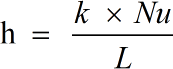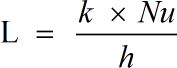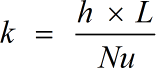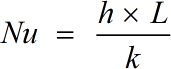Request a Tool

Nusselt Number Calculator

To calculate nusselt number enter values in given input box by using this nusselt number calculator.

Output

Heat Transfer Coefficient
0

Formula• h = Convection Heat Transfer Coefficient
• L = Characteristic Length
• k = Thermal Conductivity of the Fluid
• Nu = Nusselt Number

Defination / Uses

The Nusselt Number is a dimensionless number used in mass transfer research. The symbol 'Nu' stands for the Nusselt Number.

Output

Characteristic Length
0

Formula• L = Characteristic Length
• h = Convection Heat Transfer Coefficient
• k = Thermal Conductivity of the Fluid
• Nu = Nusselt Number

Defination / Uses

The Nusselt Number is a dimensionless number used in mass transfer research. The symbol 'Nu' stands for the Nusselt Number.

Output

Thermal Conductivity
0

Formula• k = Thermal Conductivity of the Fluid
• h = Convection Heat Transfer Coefficient
• L = Characteristic Length
• Nu = Nusselt Number

Defination / Uses

The Nusselt Number is a dimensionless number used in mass transfer research. The symbol 'Nu' stands for the Nusselt Number.

Nusselt Number
0

Formula• Nu = Nusselt Number
• h = Convection Heat Transfer Coefficient
• L = Characteristic Length
• k = Thermal Conductivity of the Fluid

Defination / Uses

The Nusselt Number is a dimensionless number used in mass transfer research. The symbol 'Nu' stands for the Nusselt Number.

How to use nusselt number calculator?

There are only two basic steps.

• First select the option from dropdown. What you need to calculate.
• Then add the other values in their corresponding fields.

Thats it! You will get your answer in less than a second. Use upper given formula in case of manual calculation.

The Nusselt number is the proportion of convective heat transfer to conductive heat transfer over a boundary. Using several formulas, this complex online Nusselt Number Calculator calculates the various parameters of Nusselt Number. The Nusselt number is a dimensionless parameter that is used in heat transfer calculations between a flowing fluid and a solid body. A boundary layer, such as the bounding surface in the interior of a pipe, is an area where these forces change behaviour. For quick calculation use our weetools. No sign-up, registration OR captcha is required to use this tool.